Next: Ground truth: verifying the Up: Estimation with unknown exposure Previous: The general case: multiple

### Weighting the solution by the certainty function

It is expected that pixel values near the middle of their range in the image will change more rapidly for a given change in exposure than pixel values close to the extremes. For example, when a pixel is saturated (e.g. 255 in the case of an eight bit camera) no additional light will change the pixel value. Therefore, we expect that the midtones in the image will give greater certainty in estimating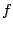than will the shadows and highlights. This intuition is made formal by the so-called certainty functionsmannwyckoff, which are the derivatives of the response functions.

The certainty functions may therefore be used to weight the columns of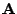, and the corresponding entries of the vector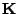, when solving for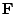: A_w=A (w1)
A_w^TA_wF - A_w^TK_w = 0 where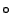denotes Hadamard multiplication5,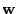is a column vector in which each entry is made up of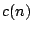where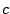is the certainty function, and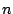is the column index in which the quantity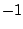appears for that row.

In practice, we do not know the response function (this is the very entity we are trying to estimate) so we also do not know a-priori, its derivative, the certainty function, which we need to use in the weighting.However, we can use a certainty function that has the general shape of the derivative of a typical response function. It has been found that the solution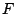is not particularly sensitive to the shape of the certainty function. A Gaussian weighting is generally used. If desired, onceis found,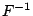can be differentiated and used as the certainty function to weight the columns ofto re-estimate. This procedure can be repeated again (e.g. giving rise to an iterative estimation of).Next: Ground truth: verifying the Up: Estimation with unknown exposure Previous: The general case: multiple
Steve Mann 2002-05-25